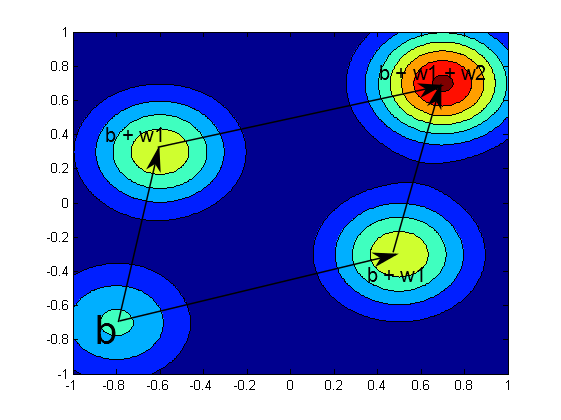1 view

I want to have a nitty-gritty understanding of Restricted Boltzmann Machines with continuous input variables. I am trying to devise the most trivial possible example so that the behavior could be easily tracked. So, here it is.

The input data is two-dimensional. Each data point is drawn from one of two symmetrical normal distributions (sigma = 0.03), whose centers are well spaced (15 times sigma). The RBM has a two-dimensional hidden layer.

I expected to obtain an RBM that would generate two clouds of points with the same means as in my train data. I was even thinking that after adding some sparsity constraints I would have the hidden layer equal to (0,1) for the data drawn from one distribution and (1,0) for the other.

I wrote MatLab code myself and tried some online solutions (such as DeepMat: https://github.com/kyunghyuncho/deepmat), but no matter how small my step size is, RBM converges to a trivial solution, in which the predicted visible layer is equal to the mean value over entire data. I tried increasing the dimensionality of the hidden layer, but it does not change anything substantially. I also tried normalizing the data by zero mean and variance - no change. I also had sigma = 1 instead of 0.03, while keeping the spread of 15*Sigma, again no change.

Since this problem is present not only in my code but also in others', I thought that I might be doing something fundamentally wrong and trying to use RBM the way the should not be used. I would appreciate comments/suggestions, or if someone could reproduce my problem.

by (108k points)

Here is an explanation of which probability density functions over visible variables can be expressed with a Gaussian-Bernoulli RBM. The following picture gives an illustration, where b is the visible bias and w1 and w2 are the weight vectors associated with the hidden units.